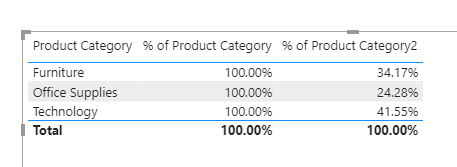Search

# DAX: Using VariablesQuestion: What is the following measure calculating?

``` Measure1 =
DIVIDE (
SUMX (
dtOrders,
dtOrders[Unit Price] * dtOrders[Order Quantity]
) - SUMX (
dtOrders, dtOrders[Unit Cost] * dtOrders[Order Quantity]
),
SUMX (
dtOrders, dtOrders[Unit Cost] * dtOrders[Order Quantity]
)
)
```

Tricky to figure it out. Isn’t it?

``` Measure 2 =
VAR _SalesAmt=
SUMX(dtOrders,dtOrders[Unit Price] * dtOrders[Order Quantity])

VAR _TotalCost =
SUMX(dtOrders,dtOrders[Unit Cost] * dtOrders[Order Quantity])

VAR _Margin =
_SalesAmt - _TotalCost

VAR _MarginPrcnt =
DIVIDE(_Margin, _TotalCost)

RETURN
_MarginPrcnt
```

Both the formula is calculating precisely the same thing. The only difference is the later requiring less attention and hence is easier to read.

We have used variables in [Measure 2]. The common purpose or usage of variables is to divide the calculation of a complex formula into logical steps, by assigning the result of each step to a variable.

## Syntax

We use the keyword VAR to define the variable, followed by the RETURN, which defines the result

``` Latest Orders =
//returns the sum of orders on the latest date

//finding latest date
VAR _MaxDate =
MAX(dtOrders[Order Date])

//calculating sum of orders on the latest date
VAR _SumLatest =
CALCULATE(
[Total Orders],
dtOrders[Order Date] = _MaxDate
)

RETURN
_SumLatest
```

As a standard writing convention, variables are usually defined at the beginning of a measure definition and then used throughout the code.

A variable can store either a scalar value or a table. In the previous example, we used VAR to store the maximum order date. In the following example, we are filtering the Orders table

``` Furniture Sales =
//filtering orders tbl for product category 'furniture'
VAR _Filter =
FILTER(
dtOrders,
dtOrders[Product Category] = "Furniture"
)

//calculate the sum of orders where product category = furniture

VAR _SumOfOrders =
SUMX(
_Filter,
dtOrders[Order Quantity]
)

RETURN
_SumOfOrders
```

When using a variable as a table, one should access the columns of a table variable by using their original names.

In the above example, we defined a variable table _Filter. In the SUMX function, we used the original column name to get the total order quantity. And, we referred to the variable table _Filter in the table argument.## Things to Remember

· We do not get VAR as an IntelliSense suggestion.All IntelliSense suggestions on VAR is for calculating variance.

• The scope of a variable is from its declaration point to the end of the expression, following the RETURN statement. A variable is never available outside its VAR/RETURN block definition.

• Referring to a variable in the measure follows a sequence. We can use the variables defined in the previous VAR statements, but not the variables defined in the following statement.

• We cannot use the variables defined in a measure into another measure.

• Despite its name, a DAX variable is a constant. It is evaluated once in the scope of the definition (VAR) and not when their value is used. For instance, the following measure returns 100% as a result

``` % of Product Category =

//calculate sales amount
VAR _SalesAmt = SUMX(dtOrders,dtOrders[Order Quantity] * dtOrders[Unit Price])

//calculate sales amount for all product category
VAR _AllOrders =
CALCULATE(
_SalesAmt,
ALL(dtOrders[Product Category])
)

//calculate % distribution
VAR _PrctContribution =
DIVIDE(_SalesAmt,_AllOrders)

RETURN
_PrctContribution

```

The following example, where we have used a measure instead of a variable:

``` Sales Amount =
SUMX(dtOrders,
dtOrders[Order Quantity] * dtOrders[Unit Price]
)

% of Product Category2 =
//calculate sales amount for all product category
VAR _AllProduct =
CALCULATE(
[Sales Amount],
ALL(dtOrders[Product Category])
)

//calculate % distribution
VAR _PrcntCalc =
DIVIDE(
[Sales Amount],  _AllProduct)

RETURN
_PrcntCalc
```

In this case, [Sales Amount] is evaluated twice; in two different filter contexts, returning two different results.Refer to the following article on calculating a percentage using DAX

## Best Practices Around Variable

• Start the name of the variables with a special character: Underscore(_). It helps in reducing the IntelliSense suggestions for the variables. Just type _ while calling the variable, and it shows a smaller list• Use a single variable as an output for RETURN. For example, in the formula above, we could write the formula DIVIDE(_Margin, _TotalCost) after RETURN. However, as a best practice, perform all calculations before RETURN and use the single variable in the result. It is useful in inspecting the formula. In case, if we want to validate the values of _Margin or _TotalCost, we can replace the variable after RETURN (RETURN _Margin or RETURN _TotalCost) to get the output, without making any changes in the formula itself.

In conclusion, variables are useful as it enhances the readability, execution, and grace of the code. Instead of writing a lengthy and complicated code, use variables to break down and simplify.

@imVivRan